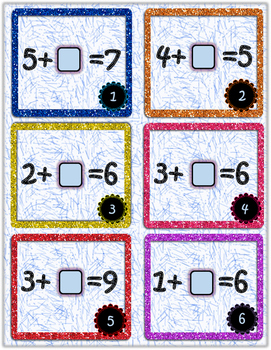Resource Type
File Type
PDF (9 MB|11 pages)
Standards
\$1.00
• Product Description
• Standards
1.OA.D.8 Determine the unknown number in an addition equation.
Determine the unknown whole number in an addition or subtraction equation relating three whole numbers. For example, determine the unknown number that makes the equation true in each of the equations 8 + ? = 11, 5 = ▯ - 3, 6 + 6 = ▯.
Total Pages
11 pages